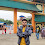# HackerRank Dynamic Array in C programming solution

In this HackerRank Dynamic array in C problem solution, there is a Snow Howler who is the librarian at the central library of the city of HuskyLand. He must handle requests which come in the following forms:

1. 1 x y: Insert a book with Y pages at the end of the Xth shelf.
2. 2 x y: Print the number of pages in the Yth book on the Xth shelf.
3. 3 x: Print the number of books on the Xth shelf.

Snow Howler has got an assistant, Oshie, provided by the Department of Education. Although inexperienced, Oshie can handle all of the queries of types 2 and 3. Help Snow Howler deal with all the queries of type 1.

## HackerRank Dynamic Array problem solution in c programming.

```#include <stdio.h>
#include <stdlib.h>

/*
* This stores the total number of books in each shelf.
*/
int* total_number_of_books;

/*
* This stores the total number of pages in each book of each shelf.
* The rows represent the shelves and the columns represent the books.
*/
int** total_number_of_pages;

int main()
{
int total_number_of_shelves;
scanf("%d", &total_number_of_shelves);

int total_number_of_queries;
scanf("%d", &total_number_of_queries);

total_number_of_books=(int*)malloc(sizeof(int)*total_number_of_shelves);

total_number_of_pages=(int**)malloc(sizeof(int*)*total_number_of_shelves);

for(int i=0; i<total_number_of_shelves; i++){
total_number_of_books[i]=0;
total_number_of_pages[i]=(int*)malloc(sizeof(int));
}

while (total_number_of_queries--) {
int type_of_query;
scanf("%d", &type_of_query);

if (type_of_query == 1) {
int x, y;
scanf("%d %d", &x, &y);
*(total_number_of_books+x)+=1;
*(total_number_of_pages+x)=realloc(*(total_number_of_pages+x), *(total_number_of_books+x)*sizeof(int));
*(*(total_number_of_pages+x)+*(total_number_of_books+x)-1)=y;

} else if (type_of_query == 2) {
int x, y;
scanf("%d %d", &x, &y);
printf("%d\n", *(*(total_number_of_pages + x) + y));
} else {
int x;
scanf("%d", &x);
printf("%d\n", *(total_number_of_books + x));
}
}

if (total_number_of_books) {
free(total_number_of_books);
}

for (int i = 0; i < total_number_of_shelves; i++) {
if (*(total_number_of_pages + i)) {
free(*(total_number_of_pages + i));
}
}

if (total_number_of_pages) {
free(total_number_of_pages);
}

return 0;
}```

1.1.